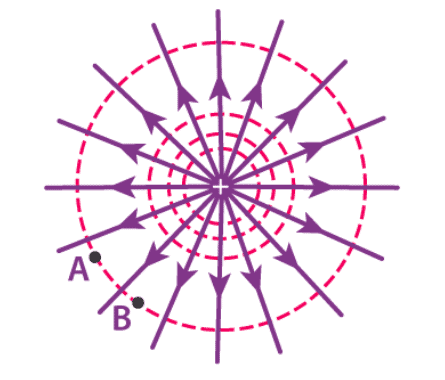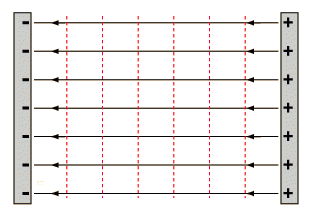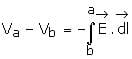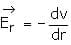NEET  >  Equipotential Surfaces

# Equipotential Surfaces Notes | Study Physics Class 12 - NEET

## Document Description: Equipotential Surfaces for NEET 2022 is part of Physics Class 12 preparation. The notes and questions for Equipotential Surfaces have been prepared according to the NEET exam syllabus. Information about Equipotential Surfaces covers topics like What is Equipotential Surface?, Equipotential Points, Work Done in Equipotential Surface and Equipotential Surfaces Example, for NEET 2022 Exam. Find important definitions, questions, notes, meanings, examples, exercises and tests below for Equipotential Surfaces.

Introduction of Equipotential Surfaces in English is available as part of our Physics Class 12 for NEET & Equipotential Surfaces in Hindi for Physics Class 12 course. Download more important topics related with notes, lectures and mock test series for NEET Exam by signing up for free. NEET: Equipotential Surfaces Notes | Study Physics Class 12 - NEET
 Table of contents What is Equipotential Surface? Equipotential Points Work Done in Equipotential Surface
 1 Crore+ students have signed up on EduRev. Have you?

What is Equipotential Surface?

• The surface which is the locus of all points which are at the same potential is known as the equipotential surface. No work is required to move a charge from one point to another on the equipotential surface.Equipotential Surface
• In other words, any surface with the same electric potential at every point is termed as an equipotential surface.

Equipotential Points

• If the points in an electric field are all at the same electric potential, then they are known as the equipotential points. If these points are connected by a line or a curve, it is known as an equipotential line.
• If such points lie on a surface, it is called an equipotential surface. Further, if these points are distributed throughout a space or a volume, it is known as an equipotential volume.

Work Done in Equipotential Surface

• The work done in moving a charge between two points in an equipotential surface is zero. If a point charge is moved from point VA to VB, in an equipotential surface, then the work done in moving the charge is given by:
W = q0(VA –VB)
As VA – VB is equal to zero, the total work done is W = 0.
Properties of Equipotential Surface
1. The electric field is always perpendicular to an equipotential surface.
2. Two equipotential surfaces can never intersect.
3. For a point charge, the equipotential surfaces are concentric spherical shells.
4. For a uniform electric field, the equipotential surfaces are planes normal to the x-axis
5. The direction of the equipotential surface is from high potential to low potential.
6. Inside a hollow charged spherical conductor the potential is constant. This can be treated as equipotential volume. No work is required to move a charge from the centre to the surface.
7. For an isolated point charge, the equipotential surface is a sphere. i.e. concentric spheres around the point charge are different equipotential surfaces.
8. In a uniform electric field, any plane normal to the field direction is an equipotential surface.
9. The spacing between equipotential surfaces enables us to identify regions of a strong and weak field i.e. E= −dV/dr ⇒ E ∝ 1/dr
Equipotential Lines in a Constant Field
• In a conducting plate-like in a capacitor, the electric field lines are perpendicular to the plates and the equipotential lines are parallel to the plates.• The illustration below shows the electric field of a positive point charge.The electric field is fixed away from the charge and potential is positive at any set distance from the charge. If the charge moves in the direction of the electric field it will move towards the lower values of potential.
• If the charge moves towards the direction opposite to that of electric field we move towards the higher values of potential.
• The illustration below shows the electric field of a point negative charge.
• Limited distance is negative at any point from the charge. If the charge moves toward the direction of the electric field and in the direction of decreasing U thus, becoming move negative. If a charge moves in the direction opposite to electric field, the increasing value of V thus, become less negative.
• Hence, the moving with the direction of electric field means moving in the direction of decreasing V and moving against the direction of electric field means moving in the direction of increasing V. Therefore, potential difference canbe expressed in terms of electric field as:• Electric field (E) as a function of potential can be expressed aswhere Er is the component of electric field along the direction of dv/dr is known as the potential gradient and the negative sign infers that electric field acts in a direction of decrease of potential.
• The expression above indicates that E is not certainly zero if V is zero.
The document Equipotential Surfaces Notes | Study Physics Class 12 - NEET is a part of the NEET Course Physics Class 12.
All you need of NEET at this link: NEET

## Physics Class 12

157 videos|425 docs|213 tests
 Use Code STAYHOME200 and get INR 200 additional OFF

## Physics Class 12

157 videos|425 docs|213 tests

### How to Prepare for NEET

Read our guide to prepare for NEET which is created by Toppers & the best Teachers

Track your progress, build streaks, highlight & save important lessons and more!

,

,

,

,

,

,

,

,

,

,

,

,

,

,

,

,

,

,

,

,

,

;